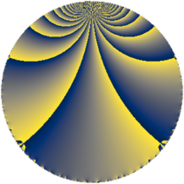# Properties

 Label 144.3.jLevel $144$ Weight $3$ Character orbit 144.j Rep. character $\chi_{144}(53,\cdot)$ Character field $\Q(\zeta_{4})$ Dimension $32$ Newform subspaces $1$ Sturm bound $72$ Trace bound $0$

# Related objects

## Defining parameters

 Level: $$N$$ $$=$$ $$144 = 2^{4} \cdot 3^{2}$$ Weight: $$k$$ $$=$$ $$3$$ Character orbit: $$[\chi]$$ $$=$$ 144.j (of order $$4$$ and degree $$2$$) Character conductor: $$\operatorname{cond}(\chi)$$ $$=$$ $$48$$ Character field: $$\Q(i)$$ Newform subspaces: $$1$$ Sturm bound: $$72$$ Trace bound: $$0$$

## Dimensions

The following table gives the dimensions of various subspaces of $$M_{3}(144, [\chi])$$.

Total New Old
Modular forms 104 32 72
Cusp forms 88 32 56
Eisenstein series 16 0 16

## Trace form

 $$32q + O(q^{10})$$ $$32q + 40q^{10} + 48q^{16} + 64q^{19} - 88q^{22} - 120q^{28} - 248q^{34} - 184q^{40} + 128q^{43} + 24q^{46} - 224q^{49} + 632q^{52} + 456q^{58} + 64q^{61} - 48q^{64} - 64q^{67} - 312q^{70} - 576q^{76} - 512q^{79} - 720q^{82} + 320q^{85} - 400q^{88} - 192q^{91} + 696q^{94} + O(q^{100})$$

## Decomposition of $$S_{3}^{\mathrm{new}}(144, [\chi])$$ into newform subspaces

Label Dim. $$A$$ Field CM Traces $q$-expansion
$$a_2$$ $$a_3$$ $$a_5$$ $$a_7$$
144.3.j.a $$32$$ $$3.924$$ None $$0$$ $$0$$ $$0$$ $$0$$

## Decomposition of $$S_{3}^{\mathrm{old}}(144, [\chi])$$ into lower level spaces

$$S_{3}^{\mathrm{old}}(144, [\chi]) \cong$$ $$S_{3}^{\mathrm{new}}(48, [\chi])$$$$^{\oplus 2}$$